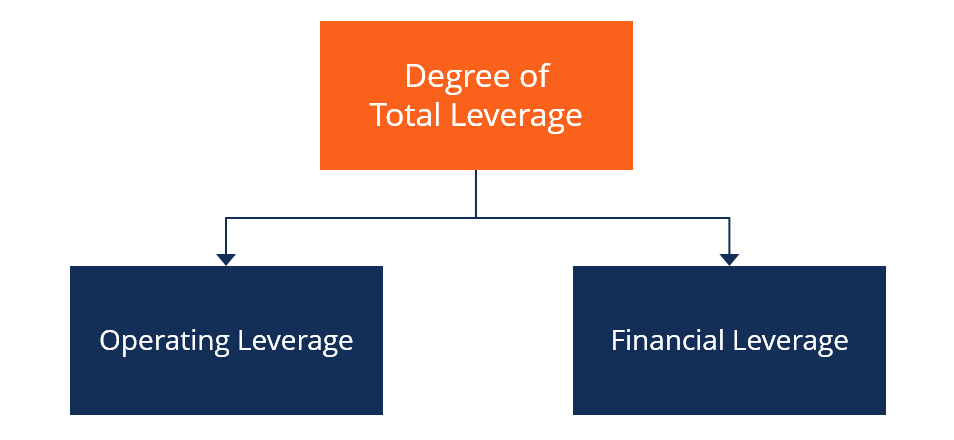# Degree of Total Leverage

The ratio between the rate of change in the company's EPS and rate of change in its revenue

## What is the Degree of Total Leverage?

The degree of total leverage is a ratio that compares the rate of change a company experiences in earnings per share (EPS) to the rate of change it experiences in revenue from sales.

The degree of total leverage can also be referred to as the “degree of combined leverage” because it considers the effects of both operating leverage and financial leverage.### Components of the Degree of Total Leverage

The two leverages that degree of total leverage accounts for are:

1. Operating leverage – This part of a company’s fixed costs reveals how effectively revenue from sales is translated into operating income. A business with a high level of operating leverage can increase its bottom line significantly with just a relatively small increase in revenues because it has effectively leveraged its operating costs so as to maximize profits.
2. Financial leverage – Financial leverage is a metric used to evaluate the extent to which a company uses debt to increase its assets and net income. Examining a company’s financial leverage shows the impact on earnings per share of changes in EBIT that result from taking on additional debt.

### Calculating Degree of Total Leverage

The degree of total leverage can be explained or calculated simply as:

Degree of total leverage = Degree of operating leverage x Degree of financial leverage =

The degree of operating leverage is equivalent to:

Contribution margin (Total sales – Variable costs) / Earnings before interest and taxes (EBIT)

The degree of financial leverage is equivalent to:

Earnings before interest and taxes (EBIT) / EBIT, less interest expenses

### Example of Degree of Total Leverage

Let’s put the degree of total leverage into practice by looking at an example. Assume that Company ABC’s current EPS is \$3, and it is trying to determine what its new EPS will be in the event that it experiences a 10% increase in sales revenue.

For our example, also assume the following for Company ABC:

• Contribution margin is \$15 million
• Fixed costs are \$3 million
• Interest expenses are \$1.5 million

The first step in determining Company ABC’s new EPS is to calculate the percentage of response that the current EPS will experience with a 1% change in revenue from sales, which is also equal to the degree of operating leverage. The calculation should look something like this:

\$15m/\$15m – \$3m (1.25) x \$15m – \$3m / \$15m – \$3m – \$1.5m (1.14) =

1.25 x 1.14 = 1.43%

The degree of total leverage for Company ABC is 1.43%. The figure can then be used to help the company determine what its new EPS will be if it sees a 10% increase in sales revenue. The calculation for the new EPS should look like this:

\$3 (current EPS) x (1 + 1.43 x 10%) = \$3.49

### Final Word

Determining a company’s degree of total leverage is important because it helps the company establish the type or percentage of change it can expect in its EPS in relation to an increase in sales revenue in relation to debt. This is important be

Understanding the changes or growth in earnings per share is important for any company because it helps corporate management evaluate the company’s performance and because it shows the income the company is earning for its shareholders.

We hope you’ve enjoyed reading CFI’s explanation of the degree of total leverage. CFI offers the Financial Modeling & Valuation Analyst (FMVA)™ certification program for those looking to take their careers to the next level. To keep learning and advancing your career, the following resources will be helpful:

• Coverage Ratio
• EV/EBITDA
• Leverage Ratios
• Types of Valuation Multiples

### Financial Analyst Training

Get world-class financial training with CFI’s online certified financial analyst training program!

Gain the confidence you need to move up the ladder in a high powered corporate finance career path.

Learn financial modeling and valuation in Excel the easy way, with step-by-step training.